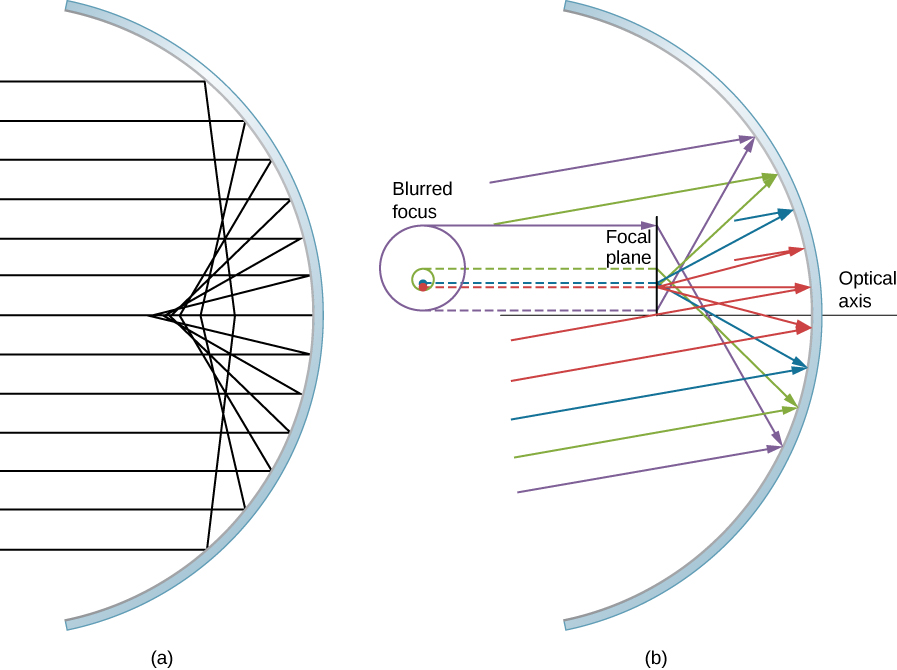# 2.2 Spherical mirrors  (Page 7/20)

 Page 7 / 20

## Problem-solving strategy: spherical mirrors

Step 1. First make sure that image formation by a spherical mirror is involved.

Step 2. Determine whether ray tracing, the mirror equation, or both are required. A sketch is very useful even if ray tracing is not specifically required by the problem. Write symbols and known values on the sketch.

Step 3. Identify exactly what needs to be determined in the problem (identify the unknowns).

Step 4. Make a list of what is given or can be inferred from the problem as stated (identify the knowns).

Step 5. If ray tracing is required, use the ray-tracing rules listed near the beginning of this section.

Step 6. Most quantitative problems require using the mirror equation. Use the examples as guides for using the mirror equation.

Step 7. Check to see whether the answer makes sense. Do the signs of object distance, image distance, and focal length correspond with what is expected from ray tracing? Is the sign of the magnification correct? Are the object and image distances reasonable?

## Departure from the small-angle approximation

The small-angle approximation is a cornerstone of the above discussion of image formation by a spherical mirror. When this approximation is violated, then the image created by a spherical mirror becomes distorted. Such distortion is called aberration    . Here we briefly discuss two specific types of aberrations: spherical aberration and coma.

## Spherical aberration

Consider a broad beam of parallel rays impinging on a spherical mirror, as shown in [link] .(a) With spherical aberration, the rays that are farther from the optical axis and the rays that are closer to the optical axis are focused at different points. Notice that the aberration gets worse for rays farther from the optical axis. (b) For comatic aberration, parallel rays that are not parallel to the optical axis are focused at different heights and at different focal lengths, so the image contains a “tail” like a comet (which is “coma” in Latin). Note that the colored rays are only to facilitate viewing; the colors do not indicate the color of the light.

The farther from the optical axis the rays strike, the worse the spherical mirror approximates a parabolic mirror. Thus, these rays are not focused at the same point as rays that are near the optical axis, as shown in the figure. Because of spherical aberration    , the image of an extended object in a spherical mirror will be blurred. Spherical aberrations are characteristic of the mirrors and lenses that we consider in the following section of this chapter (more sophisticated mirrors and lenses are needed to eliminate spherical aberrations).

## Coma or comatic aberration

Coma is similar to spherical aberration, but arises when the incoming rays are not parallel to the optical axis, as shown in part (b) of [link] . Recall that the small-angle approximation holds for spherical mirrors that are small compared to their radius. In this case, spherical mirrors are good approximations of parabolic mirrors. Parabolic mirrors focus all rays that are parallel to the optical axis at the focal point. However, parallel rays that are not parallel to the optical axis are focused at different heights and at different focal lengths, as show in part (b) of [link] . Because a spherical mirror is symmetric about the optical axis, the various colored rays in this figure create circles of the corresponding color on the focal plane.

friction ka direction Kaise pata karte hai
A twin paradox in the special theory of relativity arises due to.....? a) asymmetric of time only b) symmetric of time only c) only time
fundamental note of a vibrating string
what are matter waves? Give some examples
according to de Broglie any matter particles by attaining the higher velocity as compared to light'ill show the wave nature and equation of wave will applicable on it but in practical life people see it is impossible however it is practicaly true and possible while looking at the earth matter at far
Manikant
Mathematical expression of principle of relativity
given that the velocity v of wave depends on the tension f in the spring, it's length 'I' and it's mass 'm'. derive using dimension the equation of the wave
What is the importance of de-broglie's wavelength?
he related wave to matter
Zahid
at subatomic level wave and matter are associated. this refering to mass energy equivalence
Zahid
it is key of quantum
Manikant
how those weight effect a stable motion at equilibrium
how do I differentiate this equation- A sinwt with respect to t
just use the chain rule : let u =wt , the dy/dt = dy/du × du/dt : wA × cos(wt)
Jerry
I see my message got garbled , anyway use the chain rule with u= wt , etc...
Jerry
de broglie wave equation
vy beautiful equation
chandrasekhar
what is electro statics
when you consider systems consisting of fixed charges
Sherly
Diagram of the derive rotational analog equation of v= u+at
what is carat
a unit of weight for precious stones and pearls, now equivalent to 200 milligrams.
LoNE
a science that deals with the composition, structure, and properties of substances and with the transformations that they undergo.
LoNE
what is chemistry
what chemistry ?
Abakar
where are the mcq
ok
Giorgi
acids and bases
NavyaBy Michael SagBy OpenStaxBy Rohini AjayBy OpenStaxBy OpenStaxBy Jill ZerressenBy Richley CrapoBy OpenStaxBy OpenStaxBy Joli Julianna